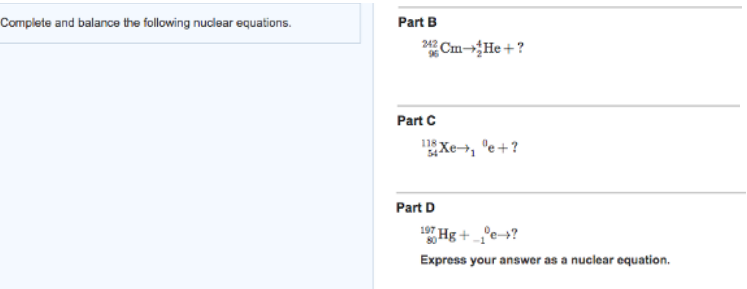# Problem: Complete and balance the following nuclear equations. Express your answer as a nuclear equation.

###### FREE Expert Solution###### Problem Details

Complete and balance the following nuclear equations. Express your answer as a nuclear equation.